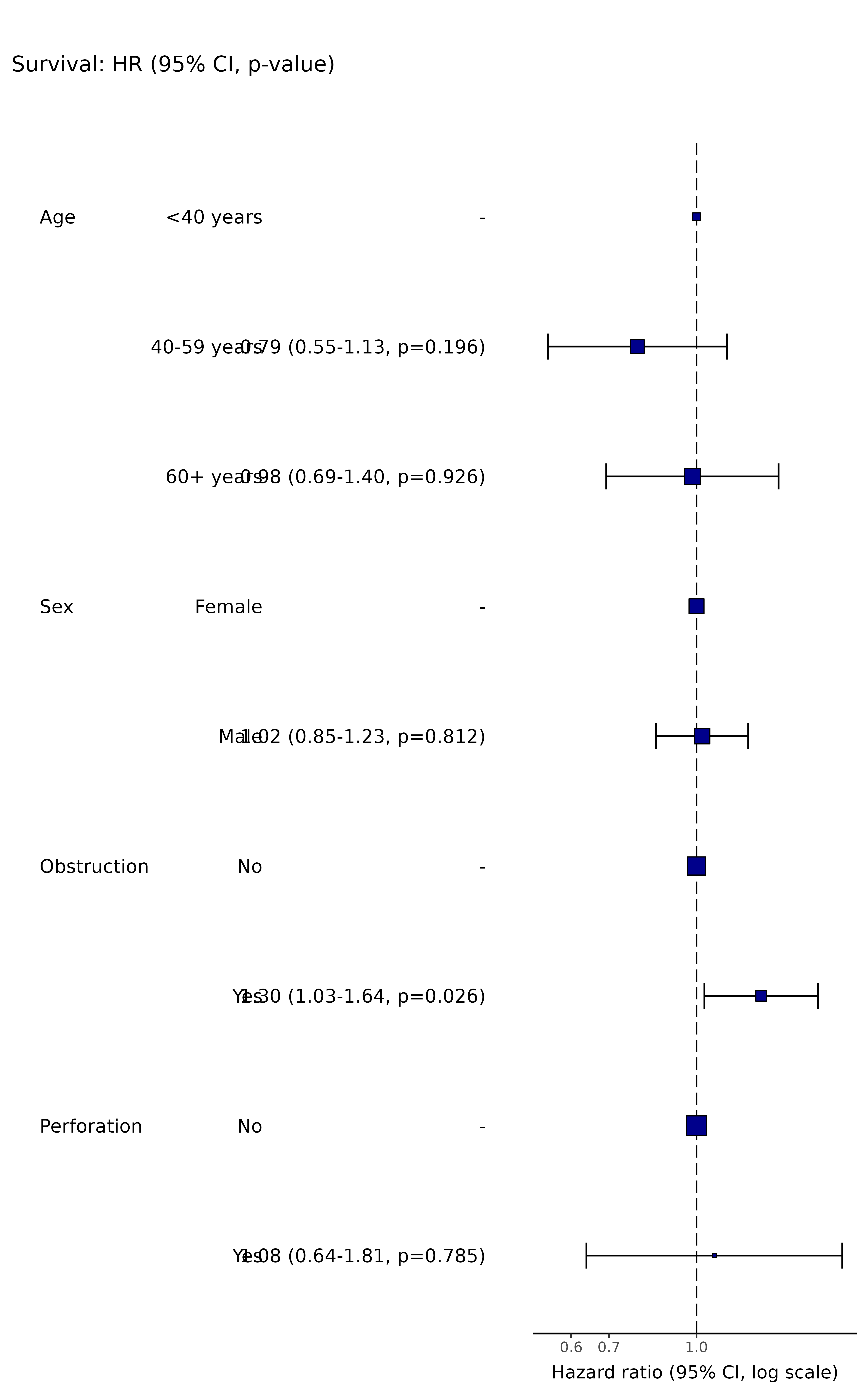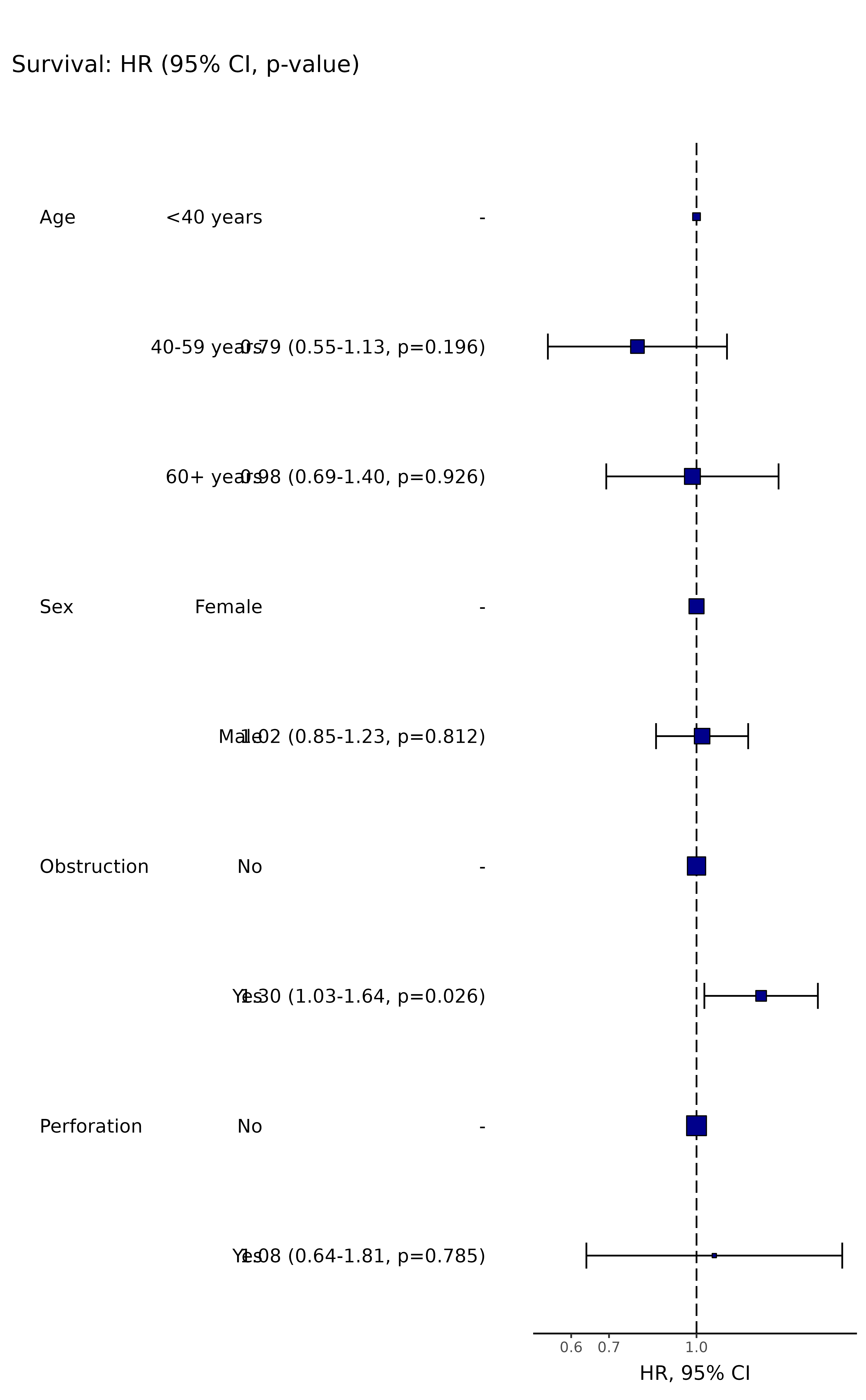Produce hazard ratio table and plot from a Cox Proportional Hazards analysis, survival::coxph().

hr_plot(
.data,
dependent,
explanatory,
factorlist = NULL,
coxfit = NULL,
remove_ref = FALSE,
breaks = NULL,
column_space = c(-0.5, 0, 0.5),
dependent_label = "Survival",
prefix = "",
suffix = ": HR (95% CI, p-value)",
table_text_size = 4,
title_text_size = 13,
plot_opts = NULL,
table_opts = NULL,
...
)

## Arguments

.data

Dataframe.

dependent

Character vector of length 1: name of survival object in form Surv(time, status).

explanatory

Character vector of any length: name(s) of explanatory variables.

factorlist

Option to provide output directly from summary_factorlist().

coxfit

Option to provide output directly from coxphmulti().

remove_ref

Logical. Remove reference level for factors.

breaks

Manually specify x-axis breaks in format c(0.1, 1, 10).

column_space

dependent_label

Main label for plot.

prefix

Plots are titled by default with the dependent variable. This adds text before that label.

suffix

Plots are titled with the dependent variable. This adds text after that label.

table_text_size

Alter font size of table text.

title_text_size

Alter font size of title text.

plot_opts

A list of arguments to be appended to the ggplot call by "+".

table_opts

A list of arguments to be appended to the ggplot table call by "+".

...

Other parameters passed to fit2df().

## Value

Returns a table and plot produced in ggplot2.

Other finalfit plot functions: coefficient_plot(), ff_plot(), or_plot(), surv_plot()

## Examples

# HR plot
library(finalfit)
library(dplyr)
library(ggplot2)

explanatory = c("age.factor", "sex.factor", "obstruct.factor", "perfor.factor")
dependent = "Surv(time, status)"
colon_s %>%
hr_plot(dependent, explanatory, dependent_label = "Survival")
#> Dependent variable is a survival object
#> Warning: Removed 4 rows containing missing values (geom_errorbarh()).colon_s %>%
hr_plot(dependent, explanatory, dependent_label = "Survival",
table_text_size=4, title_text_size=14,
plot_opts=list(xlab("HR, 95% CI"), theme(axis.title = element_text(size=12))))
#> Dependent variable is a survival object
#> Warning: Removed 4 rows containing missing values (geom_errorbarh()).